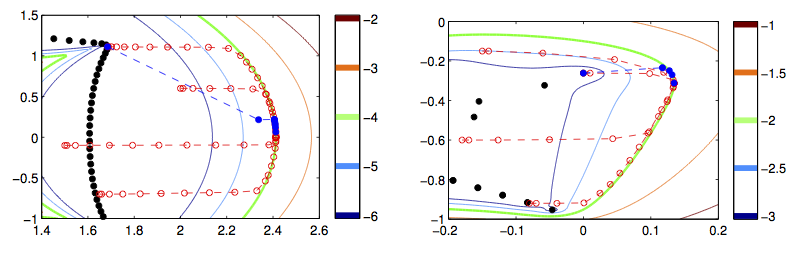# Bart Vandereycken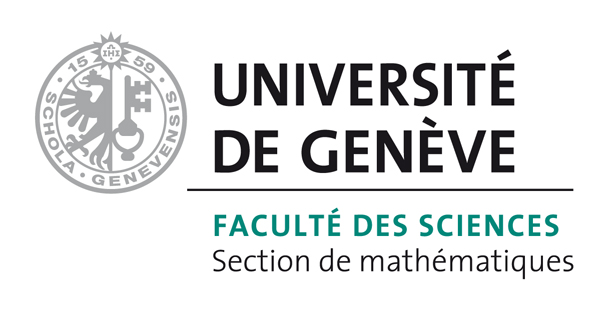## subspace_pspa: A subspace method for computing the pseudospectral abscissa.

### Content

This package contains the MATLAB code used in the paper “Subspace methods for computing the pseudospectral abscissa and the stability radius”.

### Authors

D. Kressner, EPF Lausanne
B. Vandereycken, EPF Lausanne

### Requirements

The code should work on any reasonably recent version of MATLAB. It was tested with R2011b, R2013b, R2014a (Mac OS X) and R2010b (Linux).
Although parts of the code is MEX, binary MEX files are provided so there is hopefully no need to compile them. If needed, you can try to compile them using install_mex.

### Installation

Try out example_dense, example_sparse, difficult_example_dense, difficult_example_sparse. You should get something like the following output.

```>> startup
>> example_dense
Iteration  1. Current = -7.816030706939740e-02.
Absolute error = 1.34e-04.  Relative error = 1.18e-06.
Iteration  2. Current = -7.802629355658958e-02. Relative change = 1.34e-04.
Absolute error = 3.49e-09.  Relative error = 3.09e-11.
Iteration  3. Current = -7.802629006654559e-02. Relative change = 3.49e-09.
Absolute error = 2.08e-15.  Relative error = 1.84e-17.
Iteration  4. Current = -7.802629006654559e-02. Relative change = 0.00e+00.
-- Tolerance on relative change satisfied at iteration  4. Current = -7.802629006654559e-02.
```

The code is GPLv3 licensed. In addition, it is research code and not intended for production use. If you publish a paper using this code, a reference to the above paper would be appreciated.
The code contains parts of PROPACK, EigTool, the criss-cross algorithm, PSAPSR, and eigsplus all of which may have their own licenses.

### Contact

All comments, bugs and requests are most welcome at bart.vandereycken@unige.ch.

### Version history

• v0.3 (5 October 2014): Bug fix for subspace_pspa.m not returning and displaying the most up to date approximations (thanks to Tim Mitchell, NYU). New debugging functionality to store intermediate computations and plot the iterates during the computation.

• v0.2 (26 November 2013): Bug fix for eigsplus.m in MATLAB versions R2013a and R2013b (thanks to Tim Mitchell, NYU).

• v0.1 (14 March 2012): Initial release.

### Obligatory marketing picture 1

The rectangular pseudospectra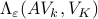of the subspaces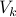generated by subspace_pspa for iterations(from left to right, top to bottom) applied to the Grcar matrix for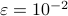. In addition, the thin lines show the square pseudospectra. See plot_grcar_pspas.m in the examples directory to produce similar figures.### Obligatory marketing picture 2

Iterates of subspace_pspa using options.tol_svd=-1 (blue discs, always eigenvalue computations) and options.tol_svd=Inf (red circles, no eigenvalue computations) for the Grcar (left figure) and the Orr-Sommerfeld (right figure) matrices of size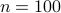. The default options.tol_svd=0.1 only performs 2 to 3 eigenvalue computations (not shown). See plot_grcar_paths.m in the examples directory to produce similar figures.